Concepts

Class 9
Chapter 10 Class 9 - Gravitation

We know that Different equations of motions are

v = u + at

s = ut + 1/2 at 2

v 2 - u 2 = 2as

where

u = Initial Velocity

v = Final Velocity

a = Acceleration

t = time taken

s = distance

Now we know that

Acceleration due to gravity for free falling objects is denoted by g

Also Distance (s )in this case becomes height of object (h) from the earth

## Hence above equations can be modified as follows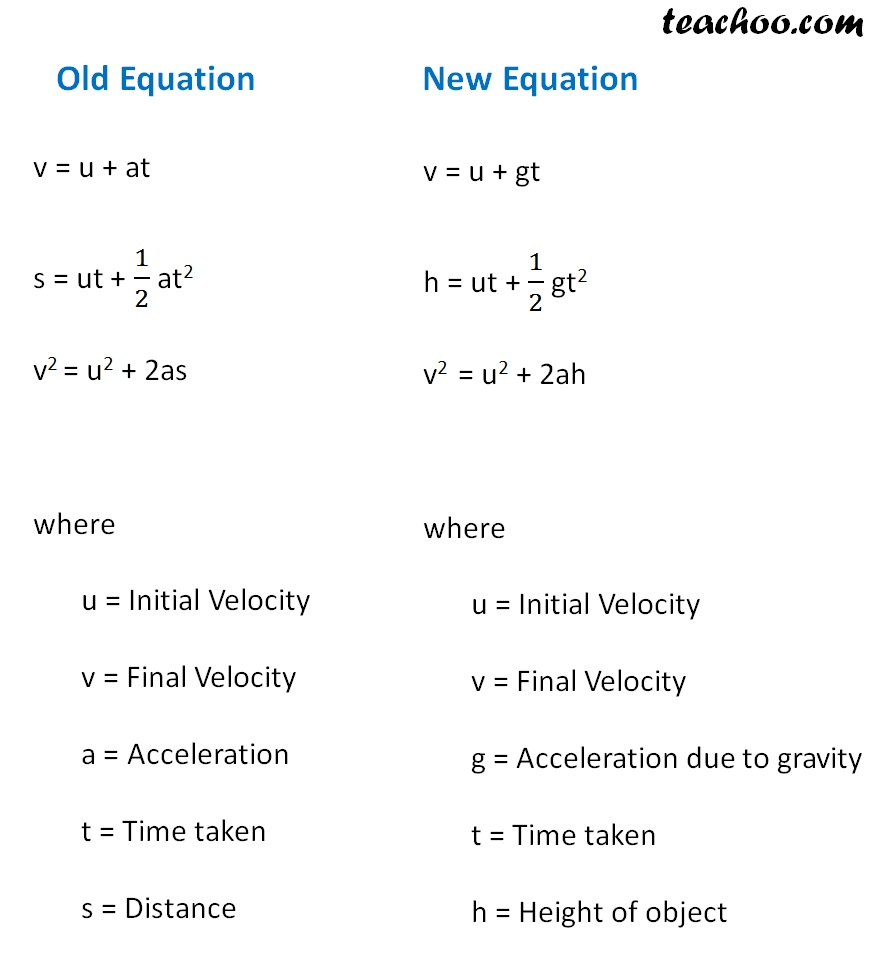To solve questions, we must remember

1. If a body is falling downwards
It's initial velocity = u = 0
and acceleration = +g = +9.8 m/s 2

2. If a body is thrown upwards
It's final velocity = velocity at highest point = 0
and acceleration = -g = -9.8 m/s 2

3. It's time of ascent = time of decent
Example - If a throw a ball upward and it takes 6 second to come down
Time taken to reach a highest point = 6/2 = 3 seconds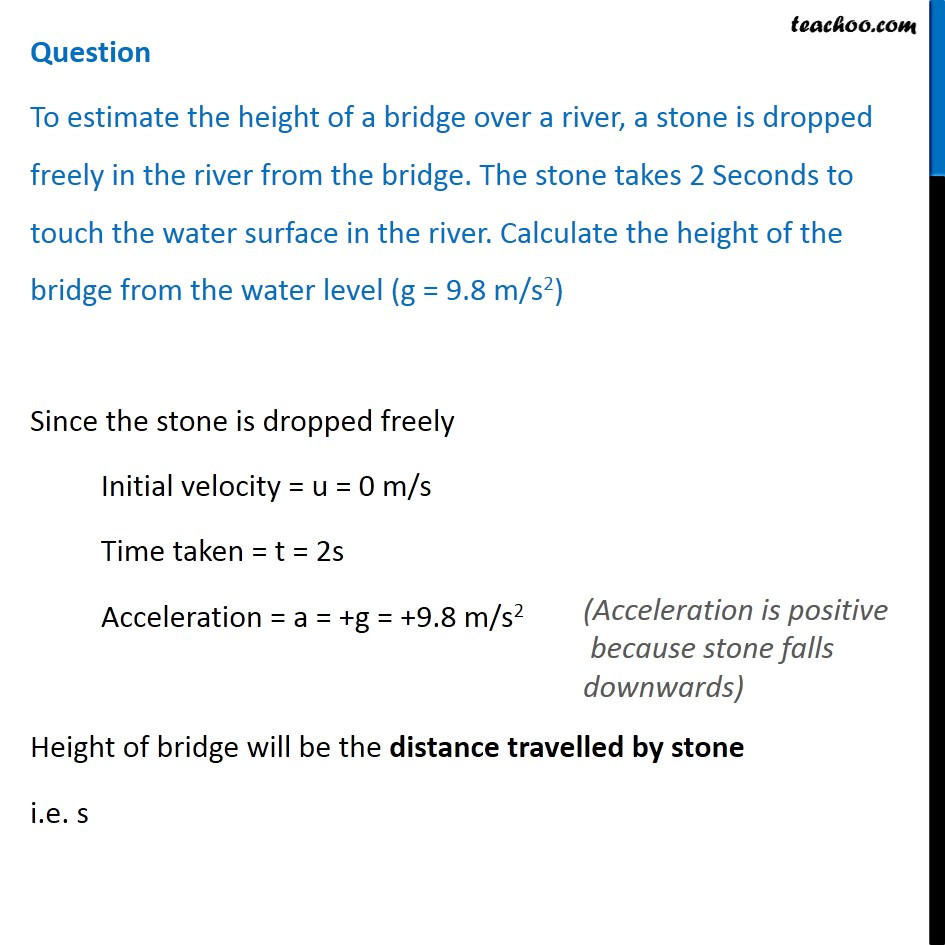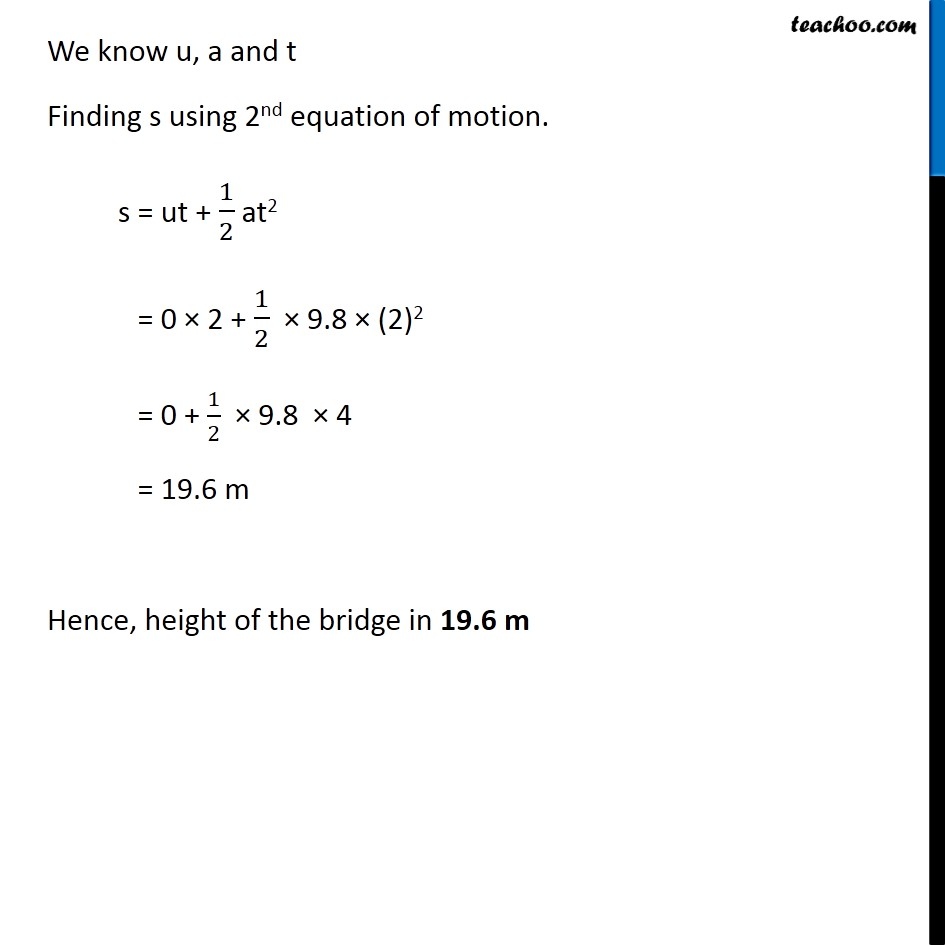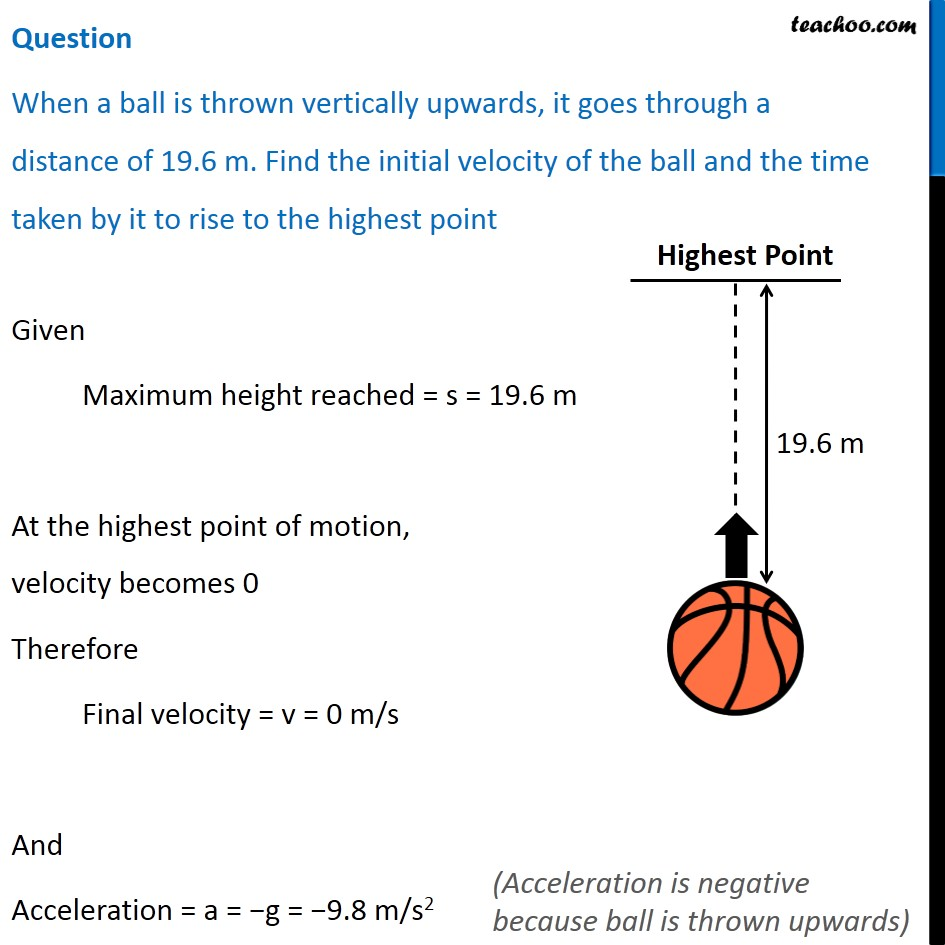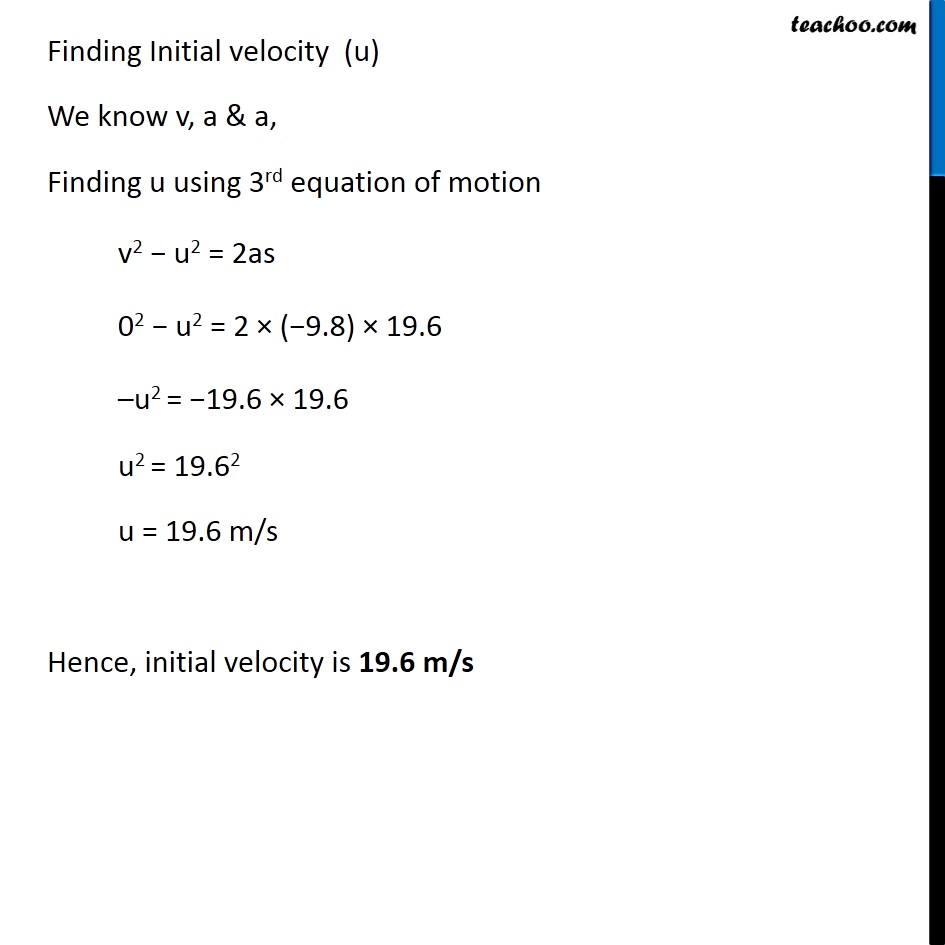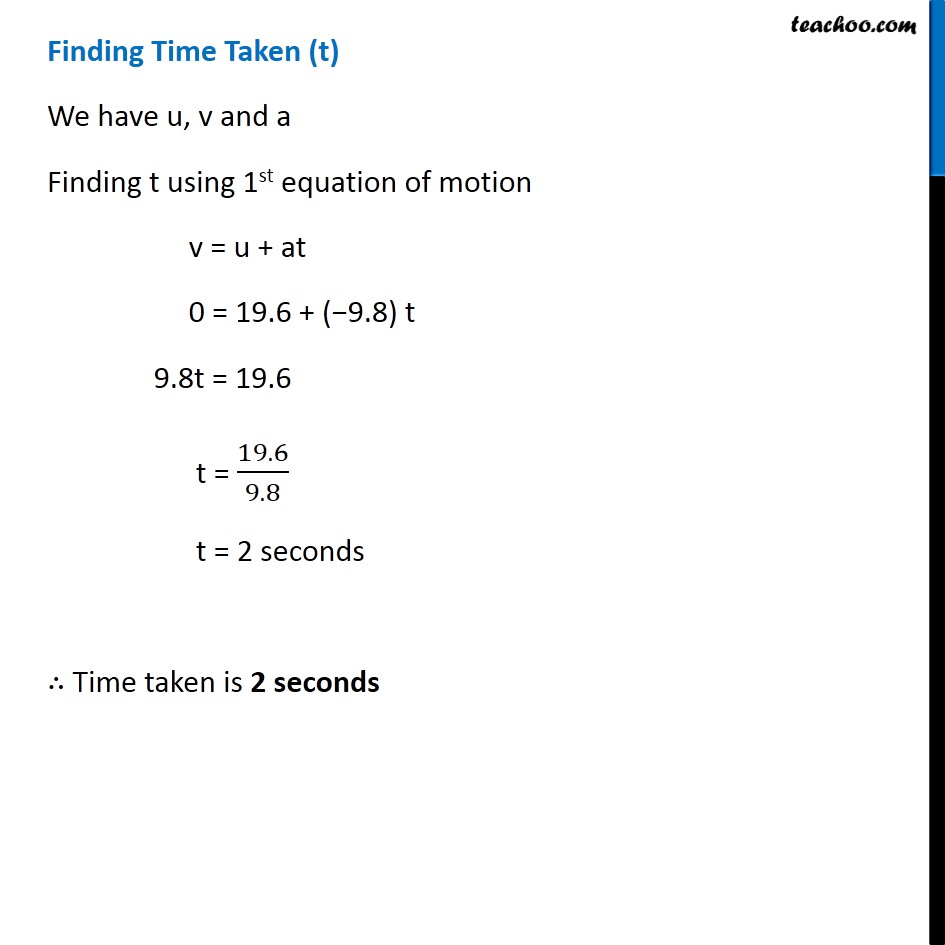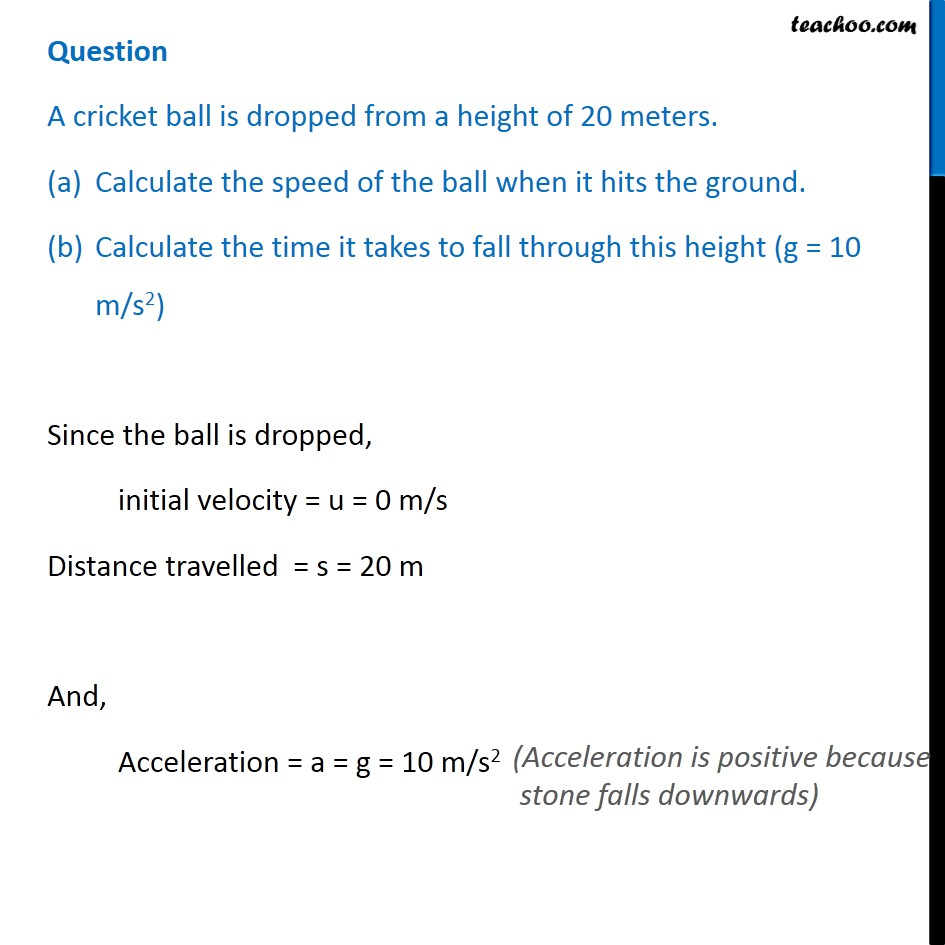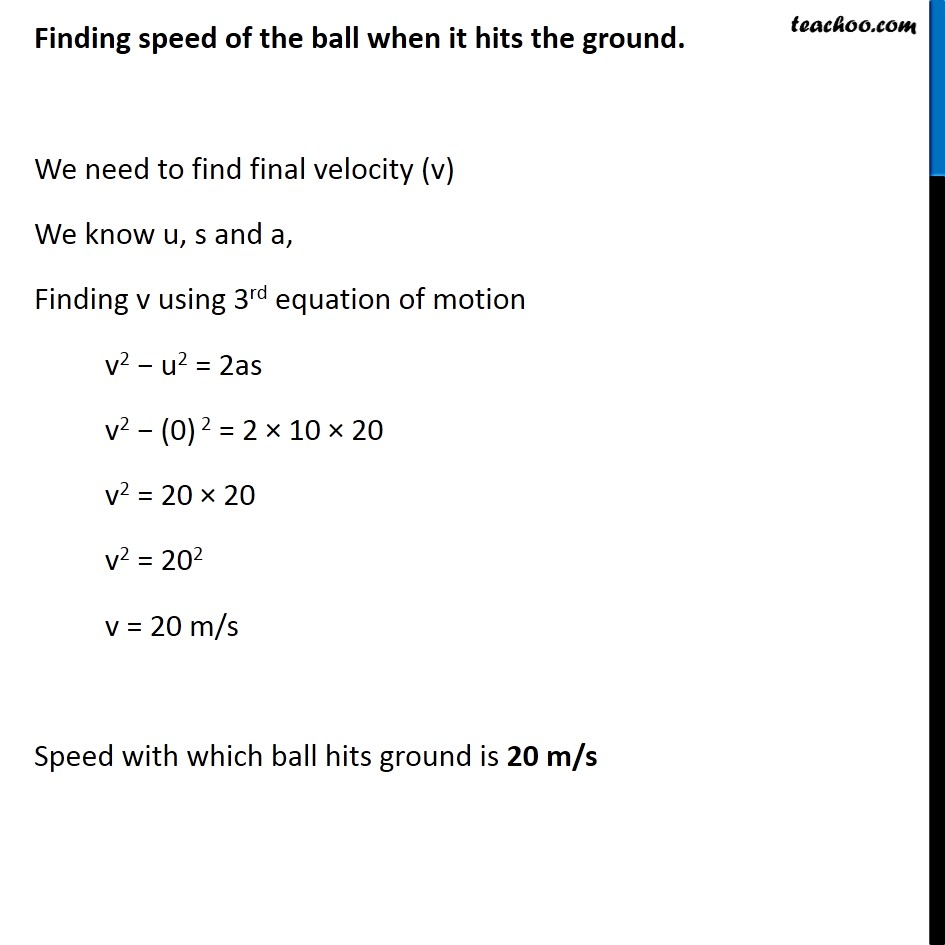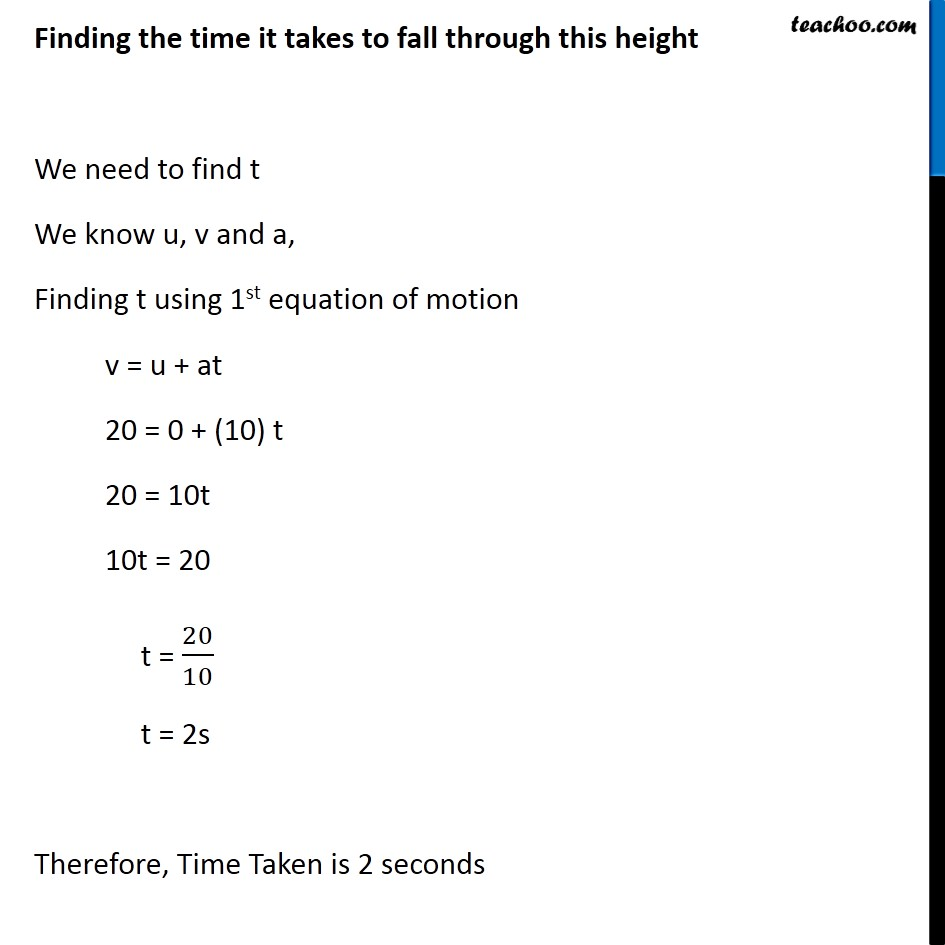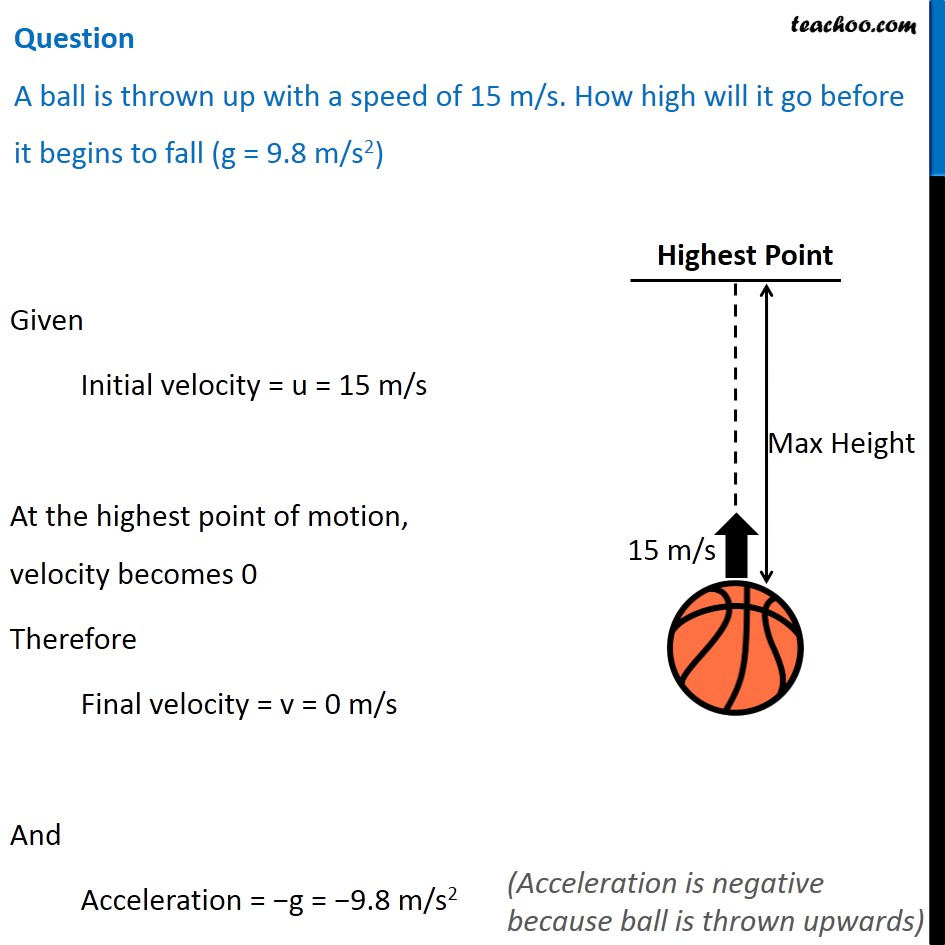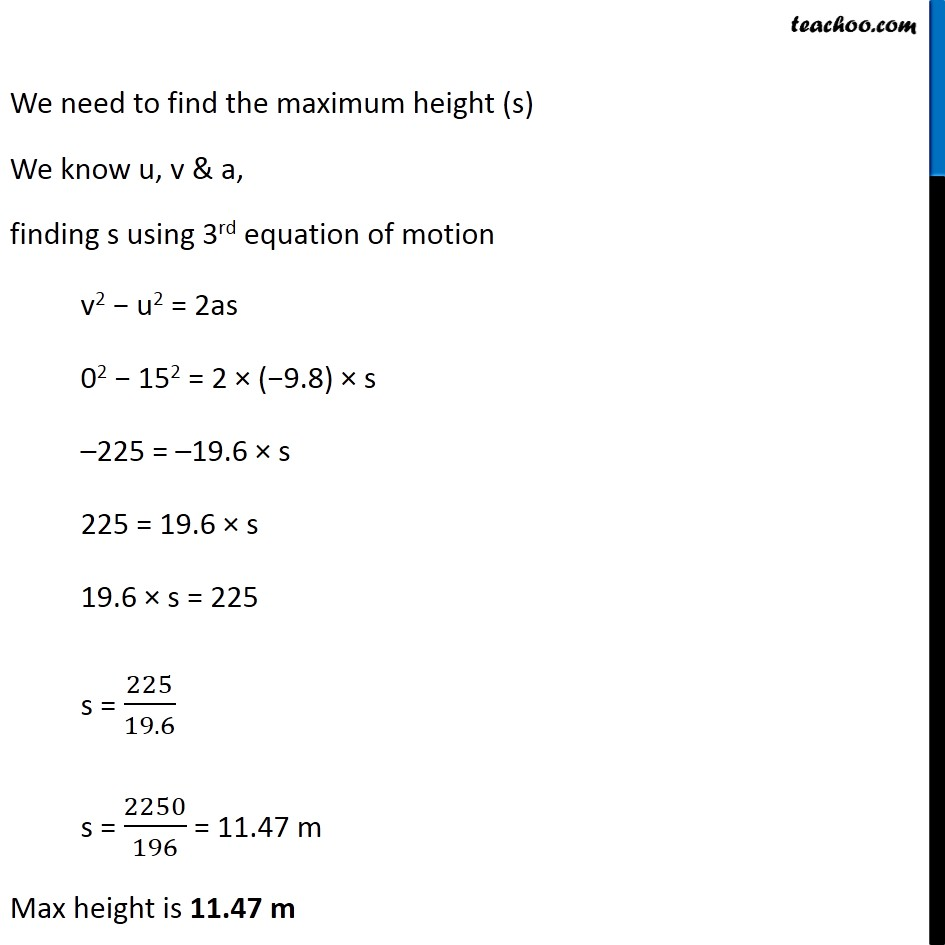## Questions

Example 10.2 - A car falls off a ledge and drops to the ground in 0.5 s. Let g = 10 m s–2 (for simplifying the calculations). (i) What is its speed on striking the ground? (ii) What is its average speed during the 0.5 s? (iii) How high is the ledge from the ground?

Example 10.3 - An object is thrown vertically upwards and rises to a height of 10 m. Calculate (i) the velocity with which the object was thrown upwards & (ii) the time taken by the object to reach the highest point

NCERT Question 13 - A ball is thrown vertically upwards with a velocity of 49 m/s. Calculate (i) the maximum height to which it rises, (ii) the total time it takes to return to the surface of the earth.

NCERT Question 14 - A stone is released from the top of a tower of height 19.6 m. Calculate its final velocity just before touching the ground.

NCERT Question 15 - A stone is thrown vertically upward with an initial velocity of 40 m/s. Taking g = 10 m/s2, find the maximum height reached by the stone. What is the net displacement & total distance covered by the stone?

NCERT Question 17 - A stone is allowed to fall from the top of a tower 100 m high and at the same time another stone is projected vertically upwards from the ground with a velocity of 25 m/s. Calculate when and where the two stones will meet.

NCERT Question 18 - A ball thrown up vertically returns to the thrower after 6 s. Find (a) the velocity with which it was thrown up, (b) the maximum height it reaches, and (c) its position after 4 s.

Learn in your speed, with individual attention - Teachoo Maths 1-on-1 Class

### Transcript

Old Equation v = u + at s = ut + 1/2 at2 v2 = u2 + 2as where u = Initial Velocity v = Final Velocity a = Acceleration t = Time taken s = Distance New Equation v = u + gt h = ut + 1/2 gt2 v2 = u2 + 2ah where u = Initial Velocity v = Final Velocity g = Acceleration due to gravity t = Time taken h = Height of object Question To estimate the height of a bridge over a river, a stone is dropped freely in the river from the bridge. The stone takes 2 Seconds to touch the water surface in the river. Calculate the height of the bridge from the water level (g = 9.8 m/s2) Since the stone is dropped freely Initial velocity = u = 0 m/s Time taken = t = 2s Acceleration = a = +g = +9.8 m/s2 Height of bridge will be the distance travelled by stone i.e. s (Acceleration is positive because stone falls downwards) We know u, a and t Finding s using 2nd equation of motion. s = ut + 1/2 at2 = 0 × 2 + 1/2 × 9.8 × (2)2 = 0 + 1/2 × 9.8 × 4 = 19.6 m Hence, height of the bridge in 19.6 m We know u, a and t Finding s using 2nd equation of motion. s = ut + 1/2 at2 = 0 × 2 + 1/2 × 9.8 × (2)2 = 0 + 1/2 × 9.8 × 4 = 19.6 m Hence, height of the bridge in 19.6 m (Acceleration is negative because ball is thrown upwards) Finding Initial velocity (u) We know v, a & a, Finding u using 3rd equation of motion v2 − u2 = 2as 02 − u2 = 2 × (−9.8) × 19.6 –u2 = −19.6 × 19.6 u2 = 19.62 u = 19.6 m/s Hence, initial velocity is 19.6 m/s Finding Time Taken (t) We have u, v and a Finding t using 1st equation of motion v = u + at 0 = 19.6 + (−9.8) t 9.8t = 19.6 t = 19.6/9.8 t = 2 seconds ∴ Time taken is 2 seconds Question A cricket ball is dropped from a height of 20 meters. Calculate the speed of the ball when it hits the ground. Calculate the time it takes to fall through this height (g = 10 m/s2) Since the ball is dropped, initial velocity = u = 0 m/s Distance travelled = s = 20 m And, Acceleration = a = g = 10 m/s2 (Acceleration is positive because stone falls downwards) Finding speed of the ball when it hits the ground. We need to find final velocity (v) We know u, s and a, Finding v using 3rd equation of motion v2 − u2 = 2as v2 − (0) 2 = 2 × 10 × 20 v2 = 20 × 20 v2 = 202 v = 20 m/s Speed with which ball hits ground is 20 m/s Finding the time it takes to fall through this height We need to find t We know u, v and a, Finding t using 1st equation of motion v = u + at 20 = 0 + (10) t 20 = 10t 10t = 20 t = 20/10 t = 2s Therefore, Time Taken is 2 seconds Question A ball is thrown up with a speed of 15 m/s. How high will it go before it begins to fall (g = 9.8 m/s2) Given Initial velocity = u = 15 m/s At the highest point of motion, velocity becomes 0 Therefore Final velocity = v = 0 m/s And Acceleration = −g = −9.8 m/s2 (Acceleration is negative because ball is thrown upwards) We need to find the maximum height (s) We know u, v & a, finding s using 3rd equation of motion v2 − u2 = 2as 02 − 152 = 2 × (−9.8) × s –225 = –19.6 × s 225 = 19.6 × s 19.6 × s = 225 s = 225/19.6 s = 2250/196 = 11.47 m Max height is 11.47 m